Beeman's algorithm

Beeman's algorithm  is is a method for numerically integrating ordinary differential equations, generally position and velocity, which is closely related to Verlet integration.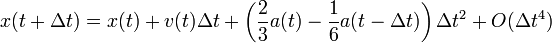$x(t+\Delta t) = x(t) + v(t) \Delta t + \left(\frac{2}{3}a(t) - \frac{1}{6} a(t - \Delta t) \right)\Delta t^2 + O( \Delta t^4)$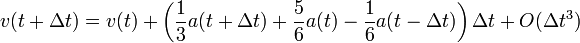$v(t + \Delta t) = v(t) + \left(\frac{1}{3}a(t + \Delta t) + \frac{5}{6}a(t) - \frac{1}{6}a(t - \Delta t) \right) \Delta t + O(\Delta t^3)$

where x is the position, v is the velocity, a is the acceleration, t is time, and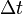$\Delta t$ is the time-step.

A predictor-corrector variant is useful when the forces are velocity-dependent:$x(t+\Delta t) = x(t) + v(t) \Delta t + \frac{2}{3}a(t) \Delta t^2 - \frac{1}{6} a(t - \Delta t) \Delta t^2 + O( \Delta t^4).$

The velocities at time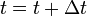$t =t + \Delta t$ are then calculated from the positions.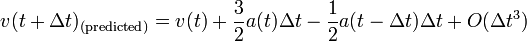$v(t + \Delta t)_{(\mathrm{predicted})} = v(t) + \frac{3}{2}a(t) \Delta t - \frac{1}{2}a(t - \Delta t) \Delta t + O( \Delta t^3)$

The accelerations at time$t =t + \Delta t$ are then calculated from the positions and predicted velocities.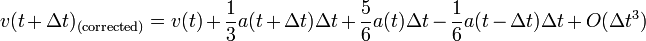$v(t + \Delta t)_{(\mathrm{corrected})} = v(t) + \frac{1}{3}a(t + \Delta t) \Delta t + \frac{5}{6}a(t) \Delta t - \frac{1}{6}a(t - \Delta t) \Delta t + O( \Delta t^3)$